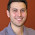## Sunday, December 27, 2009

### KD-Tree: A C++ and Boost implementation

According to wikipedia a kd-tree (short for k-dimensional tree) is a space-partitioning data structure for organiizing points in a k-dimensional space. In this implementation, points are represented as a boost ublas matrix (numPoints x dimPoints) and the kd-tree can be seen as a row permutation of the matrix. The tree is built recursively. At depth k, the (k % dimPoints) coordinates are analyzed for all the points and the median of them is selected with a quickselect algorithm. The quickselection induces a natural row-index permutation for points in two sets, which are recursively partitioned on the next levels of the tree.

Here you have the code.

#### 3 comments:

1.I can't see the code!!!

2.3.In testKdTree.cpp, the first line is:

KDtree kd (10, 10); // 30 vectors, dim=10

This is not correct. The line would need to be changed to:

KDtree kd (30, 10); // 30 vectors, dim=10

to match the comment.

David